Technical Article

# An Overview of Sequential Function Chart (SFC) PLC Programming

September 04, 2022 by Anish Devasia

## Sequential Function Chart (SFC) is a graphical programming language among the languages identified by IEC 61131-3 programming languages and standard for PLC programming using ladder logic.

SFCs are not text-based programming languages, but rather visual programming languages, similar to the flow charts for computer science algorithms.

SFCs are commonly used and easier to represent in scenarios where there are multiple states of operations.  SFC programming’s advantage is its ability to help the engineer break down large and complex processes into smaller pieces that are easy to understand.

### How Do SFC Programming Languages Work?

Sequential controls include processing sequential and parallel operations that are discrete in terms of time or events, mainly to control complex process sequences. SFC programming language makes it easier to visualize and design complex sequential systems. In this programming language, the operations are described as separate steps that are sequentially connected.

An example of this sequential connection can be seen in the operation of a washing machine. When a program is selected on the washing machine, it has multiple steps that come one after the other. When one step is over, the next automatically begins. The terms ‘steps’ and ‘state’ represent the same concept in SFC programming.

In SFC programming, states or steps are represented by rectangular boxes. A vertical straight line connects the different steps, and each step has a corresponding output. There is a transition condition between steps. Figure 1 below shows the general diagram for the sequential function chart.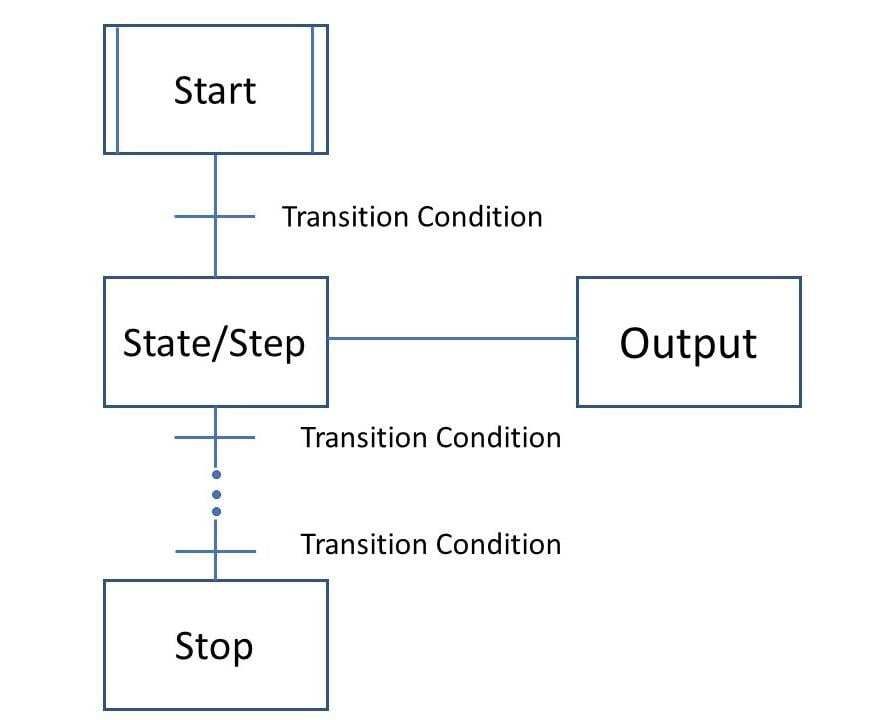##### Figure 1. SFC diagram that shows the seperate steps that are seqentially connected.

The first box represents the start of the operation. Two states are connected with a vertical line. The horizontal lines between boxes represent transition conditions. Sometimes transition conditions can be denoted by a much smaller rectangle between the larger states.

After a step is completed, the process moves on to the next state only if the transition condition(s) is also satisfied.

Each of the states also has corresponding outputs. Outputs are represented as rectangular boxes linked horizontally to their respective state.

Two conditions must be followed for every SFC:

1. There should be a transition condition between two steps.
2. A step should always separate two transition conditions.

Every SFC can be represented by an equivalent ladder logic. Figure 2 shows an example.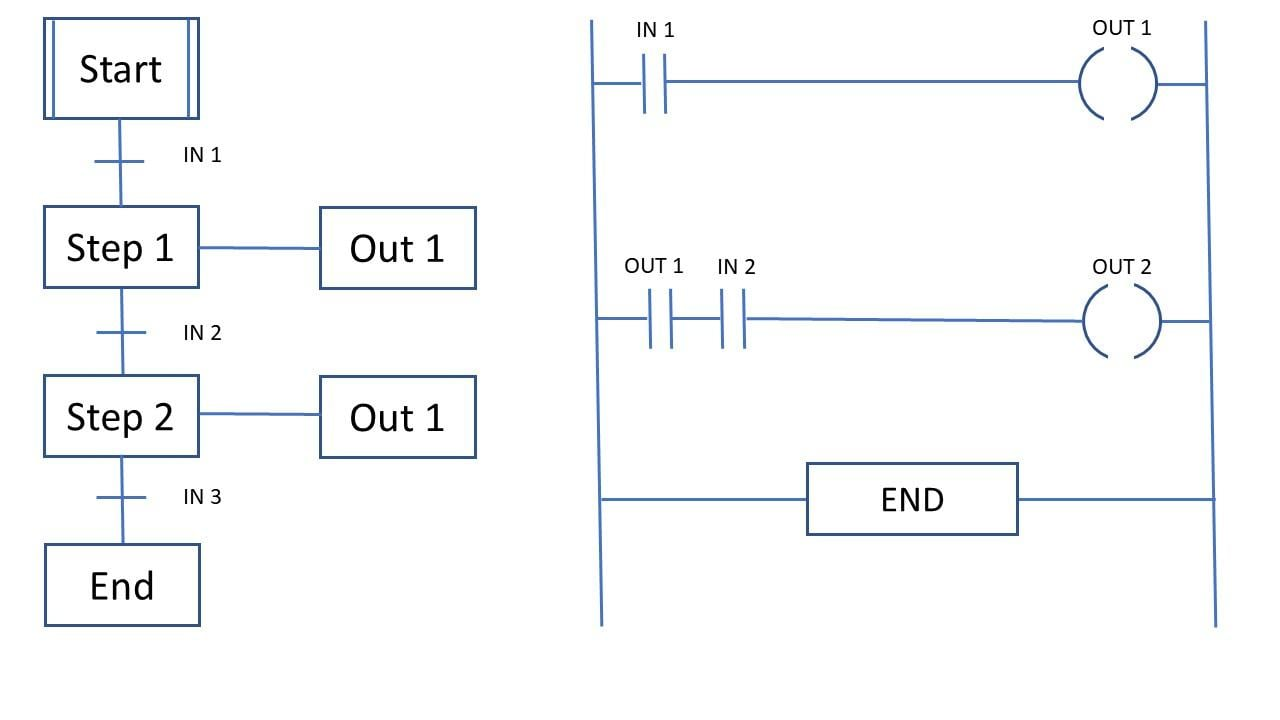##### Figure 2. SFC diagram representated by an equivalent ladder logic.

The first rung of the ladder is equivalent to Step 1. Only if IN 1 occurs will OUT 1 also activate. The second rung is equivalent to Step 2. Only if OUT 1 and IN 2 occur will OUT 2 activate.

SFCs can be summed as a combination of:

• Steps with corresponding actions
• Transitions with associated logic conditions
• Links between steps and transitions which are direct and with direction

### Program Flow

Think of the vertical lines between steps as paths from the previous state to the next state. SFC allows branching of the paths in two ways: selective and parallel. The difference between them lies in the placement of transition conditions.

#### Parallel Branches in Programming

Parallel branching allows two or more states to occur simultaneously if a single transition condition is met. In diagrams, parallel branching is represented by a pair of horizontal lines.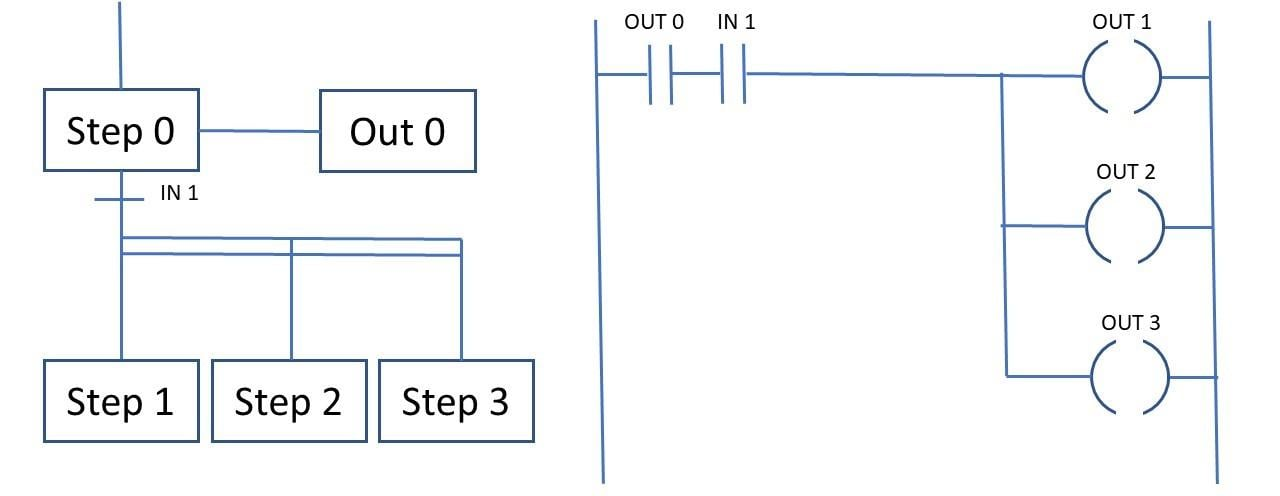##### Figure 3. Parallel branches in SFC and equivalent ladder diagram.

Once the OUT 0 is realized and the transition condition (IN 1) is met, all three steps (Step 1, Step 2, and Step 3) initiate.

#### Selective Branches in Programming

Unlike parallel branching, here not all the subsequent steps initiate simultaneously.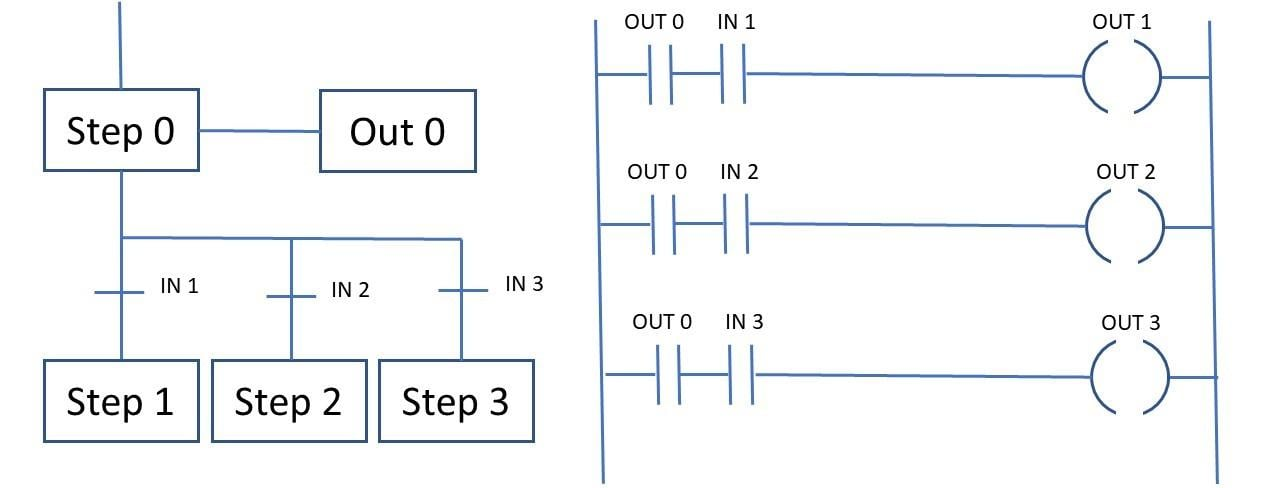##### Figure 4. Selective branches in SFC and equivalent ladder diagram.

Each step in the branch has its own transition condition that must be fulfilled. For step 1 to initiate, OUT 0 and IN 1 must occur. For step 2 to initiate, OUT 0 and IN 2 must occur. The transition condition for all the subsequent steps are independent of each other.

### SFC Programming Convergence

#### General Convergence

Similar to branching, there is also convergence possible in SFC programming. When two or more branches have a common subsequent step, it is convergence.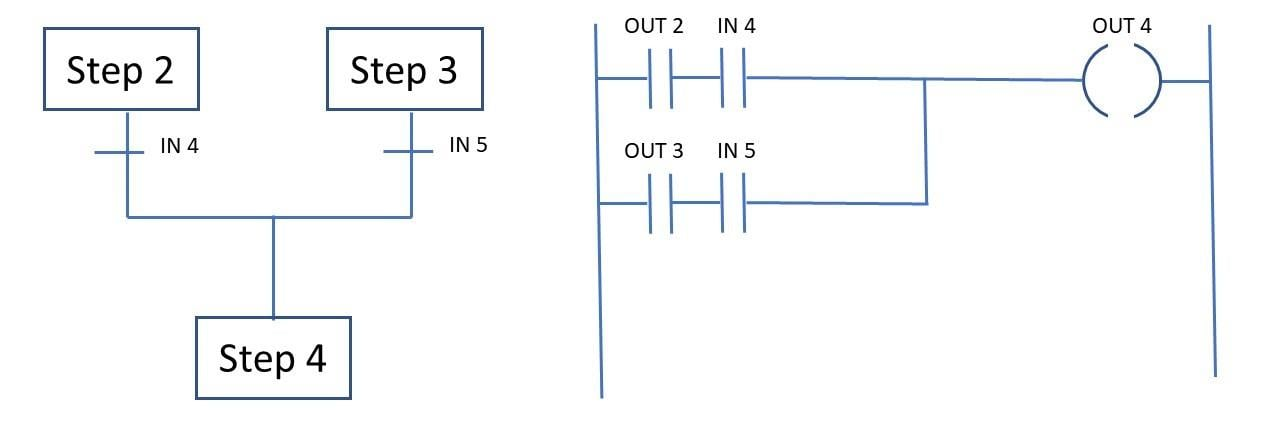##### Figure 5. Convergence in SFC and equivalent ladder diagram.

Here at least one of the two combinations must be satisfied to initiate Step 4. Either OUT 2 and IN 4 must be satisfied or OUT 3 and IN5 must be satisfied to proceed to Step 4. If all the four conditions are satisfied, it will have the same effect. If OUT and IN5 are satisfied it will not be sufficient to proceed to the next state as they are on different branches.

#### Simultaneous Convergence

The difference in simultaneous convergence compared to general convergence is that there is only one transition condition for all the previous states converging. If either one of the outputs is realized along with the single transition condition, the process moves to the next step.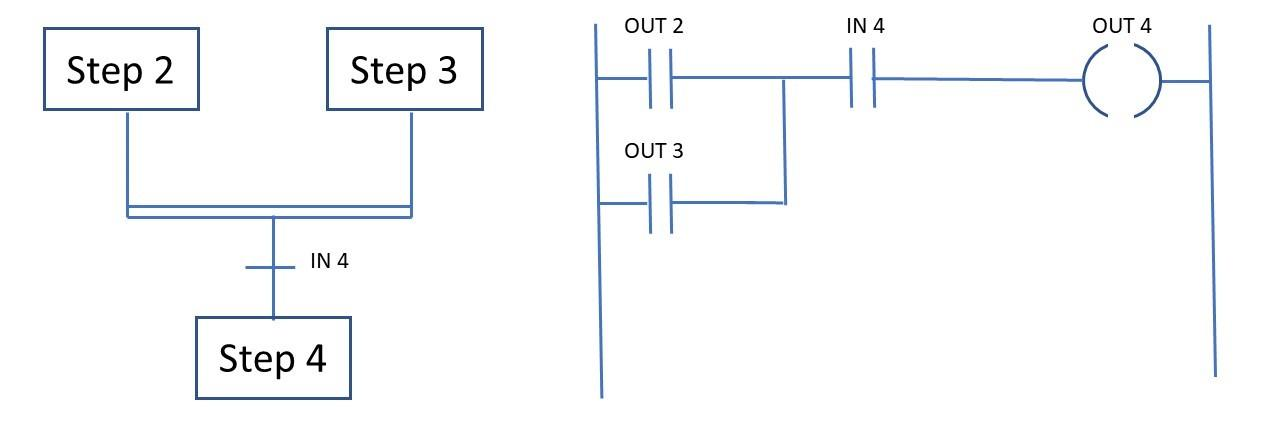##### Figure 6. Simultaneous convergence in SFC and its equivalent ladder diagram.

Figure 6 shows that there is only one transition condition for Step 2 and step 3. If either OUT 2 or OUT 3 is realized along with the transition condition IN 4, step 4 is activated.

This is an overview of the basic structure of SFCs. With this knowledge, ladder logic diagrams can be converted to sequential function charts ad vice versa. Similar conversions can be done with Functional Block Diagrams (FBD), Instruction Lists (IL), and Structured Text (ST).

1 Comment•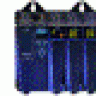pnachtwey October 01, 2020

The author didn’t say “Why bother?”
SFC are good for machine control.  SFC make it easy to organize ladder or structured text in steps or states.

SFC also makes the program more efficient because only the active step or state gets scanned instead of scanning the whole ladder.

Like.